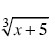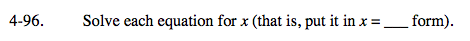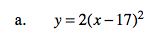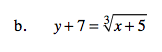### Home > A2C > Chapter 4 > Lesson 4.2.2 > Problem4-96

4-96.
1. Solve each equation for x (that is, put it in x = ____ form). Homework Help ✎

1. y = 2(x − 17)2

2. y + 7 =Divide both sides by 2.

$(\textit{x}-17)^{2}= \frac{ \textit{y} }{2 }$

Now find the square root of both sides.

$\textit{x}-17=\pm \sqrt{ \frac{ \textit{y} }{2 } }$

Now finish solving.

$\textit{x}=\pm \sqrt{ \frac{ \textit{y} }{2 } } +17$Raise both sides of the equation to the 3rd power, then subtract to isolate x. It is not necessary to expand (x + 3)3.